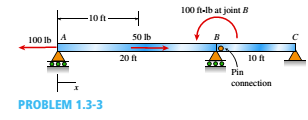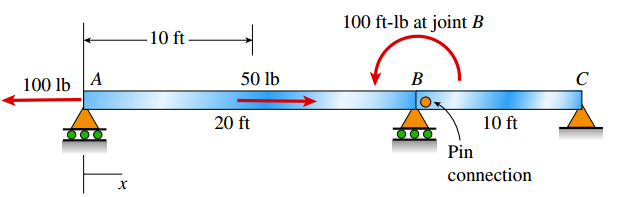Chapter 1, Problem 1.3.3PMechanics of Materials (MindTap Co...

9th Edition
Barry J. Goodno + 1 other
ISBN: 9781337093347
Textbook Problem

Segments AB and BC of beam ABC are pin connected a small distance to the right of joint B (sec figure). Axial loads act at A and at the mid-span of AB. A concentrated moment is applied at joint B. (a) Find reactions at supports A, B, and C. (b) Find internal stress resultants N, V, and M at l5 ft.To determine

You need to calculate external reaction forces at A, B and C; and the internal forces − axial force N, shear force V and bending moment M at 15 ft from A in the figure given below.Explanation

Given Information:

The following figure is given:

Calculation:

Draw free body diagram of AB section as shown in figure below for external forces,

Take equilibrium of forces in xdirection ,

Fx=0100+50+Bx=0Bx=50 lb

Now take equilibrium of moments about A,

MA=0100+By×20=0By=5 lb

Take equilibrium of forces in ydirection,

Fy=0Ay+By=0Ay=By=(5)=5Ay=5 lb

Draw free body diagram of BC section as shown in figure below for external forces,

Take equilibrium of forces in xdirection ,

Fx=0Cx=BxCx=50 lb

Take equilibrium of forces in ydirection,

Fy=0Cy=0

Now, draw free body diagram for internal reactions at 15 ft as shown in figure below,

Still sussing out bartleby?

Check out a sample textbook solution.

See a sample solution

The Solution to Your Study Problems

Bartleby provides explanations to thousands of textbook problems written by our experts, many with advanced degrees!

Get Started

Why are gases and liquids considered fluids?

Automotive Technology: A Systems Approach (MindTap Course List)

Define the term machining.

Precision Machining Technology (MindTap Course List)

What are the potential costs of implementing a database system?

Database Systems: Design, Implementation, & Management

Compute the nominal flexural strength of the composite beam in Problem 9.1-3. Use Fy=50 ksi.

Steel Design (Activate Learning with these NEW titles from Engineering!)